# MP Board Class 11th Chemistry Important Questions Chapter 6 Thermodynamics

## MP Board Class 11th Chemistry Important Questions Chapter 6 Thermodynamics

### Thermodynamics Important Questions

Thermodynamics Objective Type Questions

Question 1.

Question 1.
In adiabatic expansion of an ideal gas always:
(a) Increase in temperature
(b) ∆H = 0
(c) q = 0
(d) W = 0
(c) q = 0

Question 2.
For a reversible process, free energy change at equilibrium:
(a) More than zero
(b) Less than zero
(c) Equal to zero
(d) None of these.
(c) Equal to zero

Question 3.
In isothermal expansion of an ideal gas:
(a) 9 = 0
(b) AE = 0
(c) W = 0
(d) dV = Q
(b) AE = 0Question 4.
Hess’s law is an application of the following:
(a) First law of Thermodynamics
(b) Second law of Thermodynamics
(c) Entropy change
(d) Free energy change.
(a) First law of Thermodynamics

Question 5.
When the value of heat of neutralization of an acid with a base is 13.7 kcal, then:
(a) Acid and base both are weak
(b) Acid and base both are strong
(c) Acid is strong and base is weak
(d) Acid is weak and base is strong
(b) Acid and base both are strong

Question 2.
Fill in the blanks:

1. Enthalpy is an …………………….. property.
2. Nicely closed thermos flask is an example of an ……………………….
3. Value of heat of combustion (∆H) is always ………………………..
4. Extensive property depend on the ………………………. of matter.
5. Value of heat of neutralization is always …………………………. kilocalorie.

1. Extensive
2. Isolation
3. Negative
4. Amount
5. – 13.7Question 3.

1. Tell the value of heat of neutralization of strong acid and strong base?
2. Give two examples of state function?
3. What is entropy?
4. Among NaCl, H2O(s) and NH3(g) value of whose entropy is higher?
5. Expect the system, what is the remaining part of the universe called?
6. System in which changes occur spontaneously and by which entropy of the system increases, what are they called?
7. What is the type of heat of combustion?

1. – 57 kJ
2. Enthalpy, Entropy
3. Measure of disorder
4. NH3
5. Surroundings
6. Spontaneous process
7. Exothermic.

Thermodynamics Very Short Answer Type Questions

Question 1.
Give two examples of state function?
Enthalpy and Entropy.

Question 2.
Whose entropy is greater among NaCl, H2O(s) and NH3(g)?
NH3(g).

Question 3.
Who gave the equation ∆G = ∆H – T∆S?
Gibbs – Helmholtz.

Question 4.
Write the equation of first law of thermodynamics?
∆E = q + W.Question 5.
What is the value of entropy when ice melts?
Entropy increases.

Question 6.
What is Closed system?
System which can exchange energy only and not matter with the surroundings.

Question 7.
The value of which enthalpy is always negative?
Enthalpy of combustion.

Question 8.
Heat of neutralization of strong acid and strong base is equal to?
-13.7 kcal or -57.1 kJ.

Question 9.
A process in which no exchange of heat between system and surroundings occur is known as adiabatic process.

Question 10.
What is Enthalpy?
Heat change at constant pressure is known as enthalpy.Question 11.
The process in which pressure remains constant is called?
Isobaric process.

Question 12.
In Exothermic reaction the value of ∆H is?
Negative.

Question 13.
Unit of specific heat capacity is?
joule per kelvin per gm.

Question 14.
The relation between ∆G, ∆S and ∆H is given by?
∆G = ∆H – T∆S.

Question 15.
Which type of property is heat capacity?
Extensive property.

Question 16.
What type of properties are temperature, pressure and surface tension?
Intensive property.Question 17.
What is the unit of molar heat capacity?
joule kelvin-1 mol-1.

Question 18.
For which process dq = 0?

Question 19.
The efficiency of any fuel is measured by which value?
Calorific value.

Question 20.
What is entropy?
The measurement of degree of disorder or randomness of the molecule of the system.

Question 21.
Write the relation between standard free energy change ∆G° and equilibrium constant (K)?
∆G° = -RTlnK.Question 22.
What is the value of ∆G for Spontaneous process?
∆G < 0.

Question 23.
“The electricity obtained from an electrochemical ceil is equivalent to decrease in free energy”. Write expression for this sentence?
∆G° = -nE°F.

Thermodynamics Short Answer Type Questions – I

Question 1.
What is System?
System:
A specified portion of the universe which is selected for experimental or theoretical investigations is called the system. (MPBoardSolutions.com) In the system, the effects of certain properties such as pressure, temperature, etc. are observed. A system is said to be homogeneous if it consists of only one phase. On the other hand, it is heterogeneous if it consists of more than one phase.

Question 2.
What is process and what are its kinds?
The operation which brings about change in the state of a system is called a thermodynamics process.
Thermodynamics process may be further classified as follows:

1. Isothermal process
3. Isobaric process
4. Isochoric process
5. Reversible process
6. Irreversible process
7. Cyclic process.Question 3.
Explain Exothermic reaction with example?
Or, Explain why the value of enthalpy change negative for exothermic reaction?
The reactions in which heat is emitted are called exothermic reactions. In such reactions total heat content of products is less than that of reactants. Hence, ∆H is negative.
Example: 2NO(g) → N2(g) + O2(g); ∆H = – 180.5 kJ/mol.

Question 4.
Explain extensive and intensive properties?
1. Intensive properties:
The properties of the system which are independent of the amount of matter present in it, are called intensive properties.
Example: Temperature, viscosity, surface tension, refractive index, specific heat, density, etc.

2. Extensive properties:
The properties of the system which depend upon the amount of matter present in it, are called extensive properties.
Example: Mass, volume, energy, etc.

Question 5.
State and Explain Zeroth law of Thermodynamics?
According to this law, “Two bodies which are separately in thermal equilibrium with a third body are also in thermal equilibrium with each other”.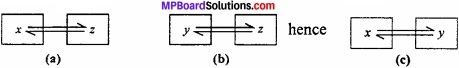Question 6.
Explain Enthalpy of neutralization with example?
The enthalpy of neutralization is defined as:
“Change in enthalpy when one gram equivalent of an acid is neutralized with one gram equivalent of base in dilute solution at constant temperature.
Example: NaOH(aq) + HCl(aq) ⇄  NaCl(aq) + H2O(l); ∆H =- 57.1 kJ

Question 7.
The enthalpy of neutralization of weak acid and weak base is less than enthalpy of neutralisation of strong acid and base. Why?
If either acid or base weak then its ionisation in solution remains incomplete. As a result a part of energy liberated during combination of H+ and OH” ion is used up for the ionisation of weak acid and weak base. Therefore, the value of enthalpy of neutralisation of weak acid with strong base or vice – versa is numerically less than – 57.1 kJ.Question 8.
What is Bond enthalpy and bond dissociation energy?
It is a well known fact that during the formation of a chemical bond, energy is required. Therefore the breaking of a bond energy is to be supplied. Thus, the energy required to break a particular bond in a gaseous molecule is called bond dissociation energy.
Example: 2HCl(g) → H2(g) + Cl2(g)

Question 9.
What is the first law of thermodynamics? Write its mathematical form?
Or, Write the first law of thermodynamics and derive the mathematical expression of it?
First law of thermodynamics is the law of conservation of energy .The common statement of this law is:
“Energy can neither be created nor be destroyed but it can be converted from one form to another form.” Let internal energy of the system is E1 and q calorie heat is supplied to the system. E2 is the energy of the final stage and work done is W. Therefore,
E2 – E1 = q + W
or ∆E = q + W.

Question 10.
Define the term Entropy?
A change that brings about disorder or randomness is more likely to occur than one that brings about order. To account for the randomness or disorder of a system a state function called entropy was introduced. It is (MPBoardSolutions.com) defined as the measure of degree of disorder or randomness of the molecule of the system. Entropy is represented by symbol S. It is easier to define entropy change than entropy of a system.Question 11.
Whose entropy is more: Water vapour, water or ice, why?
Entropy is the measure of randomness. In solid state the molecules are completely arranged, therefore its entropy is minimum and in gas the molecules move randomly in all directions, so the value of entropy is more. So
S(ice) < S(water) < S(water vapour).

Question 12.
Among NaCl, H2O and NH3 whose entropy will be maximum and why?
Entropy is the measure of randomness. In solid state the molecules are regularly arranged, so entropy is minimum whereas in gas the molecules move randomly in all directions, so the entropy is maximum. So, in the above example, NaCl is a solid, H2O is liquid and NH3 is a gas. So, the entropy of NH3 is maximum and entropy of NaCl is minimum.

Question 13.
Prove that: P∆V = ∆ntRT?
From ideal gas equation
P V = nRT
If the volume of gas at initial state is V1 and the number of moles of gas is n1 then,
PV1 = ∆n1RT …………….. (1)
If at final state, the volume of gas is V2 and number of moles of gas is n2, then,
PV2 = ∆n2RT …………. (2)
From eqn. (i) and (2),
P(V2 – V1) = (n2 – n1)RT
or P∆V = ∆nRT.

Question 14.
What is relation between ∆H and ∆U?
If H be the enthalpy of any system and U is the internal energy, then the relation will be
H = U + PV
For enthalpy change, ∆H = ∆U + P∆V
We know that P∆V = ∆nRT
On putting the value,
∆H = ∆U + ∆nRT.Question 15.
What do you mean by specific heat capacity?
It is the heat required to raise the temperature of 1 gram of substance by 1°C. It is denoted by Cs.
Cs = $$\frac{C}{m}$$
Where, C = Heat capacity, Cs = Specific heat capacity, m = Mass of substance.
Its S.I. unit is joule K-1g-1.

Question 16.
What do you mean by molar heat capacity?
It is the heat required to raise the temperature of 1 mole of substance by 1°C.
Molar heat capacity = $$\frac{C}{n}$$ $$\frac{q}{∆T × n}$$
Where C = Heat capacity (Absorbed heat), ∆T = Increase in temperature, n = Number of moles.
Its S.I. unit is joule K-1 mol-1.

Question 17.
Write Hess’s law?
In 1840, G.H. Hess formulated a law known as Hess’s law. According to law, “The enthalpy change in a physical or chemical change is same whether the process is carried out in one step or in several steps.”

Question 18.
A process in which no exchange of heat between system and surroundings occur is known as adiabatic process. This process mainly occurs in isolated system. For this type of process dq = 0.Question 19.
What is standard enthalpy of reaction?
The enthalpy change takes place at standard state i.e., at 298 K temperature (25°C), 1 atm pressure (760 mm) condition is called standard enthalpy of reaction. It is denoted by ∆H°r or ∆rH°.

Question 20.
What is enthalpy of solution? Explain with example?
Enthalpy change taking place during the dissolution of one mole of a substance in excess of a solvent such that further addition of solvent does not produce any heat change is known as enthalpy of solution.
Example: KCl(s) + aq → KCl(aq)

Question 21.
What is enthalpy of hydration? Explain with example?
Enthalpy change taking place when one mole of anhydrous salt combines with the required number of moles of water to form hydrated salt is called enthalpy of hydration.
Example: CuSO4(s) + 5H2O(l) → CuS04.5H2O ∆H = – 78.2kJ.

Thermodynamics Short Answer Type Questions – II

Question 1.
What is law of energy conservation? Write its mathematical expression. Or, What is the first law of thermodynamics? Deduce its mathematical expression?
This law was first expressed by Meyer and Helmholt. According to this law, “Energy cannot be created or destroyed although one form and vice – versa”.
Or
Whenever a certain quantity of energy in one form disappear, an equivalent amount of energy in another form reappear.

Mathematical form:
Let us suppose that system has internal energy equal to U1. If it absorbs heat energy 17 from the surroundings then internal energy will increase and becomes U1 + q. If the work is done on the system then its final internal energy will become
U2 = U1 + q + w
U2 – U1 = q + w,
∆U – q + w, (∴ U2 – U1 = ∆U
If the work done on the system is w, then
∆U = q + w
If work done by the system
∆U = q – wQuestion 2.
What is Enthalpy of fusion? Explain with example?
Enthalpy change taking place during the conversion of 1 mole of a solid substance into liquid at its melting point is known as enthalpy of fusion.
Example: Enthalpy of fusion of ice at 273 K is 6.0 kJ.
H2O(s) → H2O(l);
i.e 6.0 kJ of energy is absorbed for the conversion of 1 mole of ice into water. ∆H = +6.0 KJ

Question 3.
For an isolated system if ∆U = 0, what will be ∆S?
For an isolated system, AU = 0 and for a spontaneous process, the change in entropy should be positive. For example: For a closed container, which is an isolated system, two gases A and B are diffused (MPBoardSolutions.com) Both gases A and B are separated by a kinetic separator. When the separator is removed, the gases starts fusing with each other and randomness increases in the system. For this process, ∆S > 0 and ∆U = 0.
Again ∆S = $$\frac { q_{ rev } }{ T }$$ = $$\frac { \Delta H }{ T }$$ = $$\frac { \Delta U+P\Delta V }{ T }$$ = $$\frac { P\Delta V }{ T }$$ [∴∆U = 0]
So, T∆S or ∆S > 0.Question 4.
On the basis of the following equations, write a note on thermodynamic stability of NO(g):
$$\frac{1}{2}$$ N2(g) + $$\frac{1}{2}$$ O2(g) → NO(g); ∆rH° = 90kJ mol-1
NO(g) + $$\frac{1}{2}$$ O2(g) → NO2(g); ∆rH° = 94kJ mol-1
NO(g) is unstable, as the preparation of NO is an endothermic reaction. But preparation of NO2(g) occurs because it is an exothermic reaction (energy evolved). So unstable NO(g) is converted into NO2(g).

Question 5.
At equilibrium state, whose value will be zero ArG or ArG°?
rG = ∆rG° + RTlnK
At equilibrium, 0 = ∆rG° + RTlnK
or ∆rG° = – RTlnK
rG° = 0 (When K = 1)
For other values K, ∆rG° will be zero.

Question 6.
What is the meaning of calorific value of any fuel? Explain with example?
The heat or energy evolved in joule or calorie by the (combustion) burning of 1 gram food or fuel is known as calorific value of fuel.
C2H12O6(s) + 6O2(g) → 6CO2(g) + 6H2(g); ∆H = -2840 kJ
In this process from 1 mole glucose or 180 gm = 2480 kJ energy obtained
So, energy obtained from 1 gm glucose = $$\frac{2480}{180}$$ = 15.78 kJ/gm.
So, calorific value of glucose is 15.78 kJ/gm.

Question 7.
Explain heat of vapourization and heat of reaction?
Heat or Enthalpy of vapourization : Enthalpy change taking place during the conversion of 1 mole of liquid into vapours at its boiling point and 1 atm pressure is called enthalpy of vapourization.
Example:
H2O ⇄ H2O(g); ∆H = +40.7 kJ

Heat of reaction:
Enthalpy change taking place when number of moles of reactants as represented by the chemical equation have completely reacted is known as enthalpy of reaction. It is denoted by ∆Hf.
Example: C(s) + O2(g) → CO2(g); ∆Hf = – 393.5 kJ/molQuestion 8.
Explain enthalpy of fusion and enthalpy of sublimation with example?
Enthalpy of fusion:
Enthalpy change taking place during the conversion of I mole of a solid substance into liquid at its melting point is known as enthalpy of fusion.
H2O(s) ⇄ H2O(l); ∆H = +6.01 kJ
Enthalpy of Sublimation:
Enthalpy change taking place when one mole of solid changes into vapours without passing into intermediate liquid state at a temperature below its melting point is known as enthalpy of sublimation.
I2(s) ⇄  I2(g). ∆H = +62.4 kJ

Question 9.
How the change in entropy takes place in vapourisation process?
Entropy of vapourisation:
Change in entropy when one mole of a liquid at its boiling point changes to the vapour state at the same temperature.
∆Svapour = S(vapour) – ∆S(liquid) = $$\frac { \Delta H_{ vap } }{ T_{ b } }$$
Here, ∆vap H is the latent heat of vapourisation (enthalpy of vapourisation) and Tb is the boiling point when liquid is converted into vapour, entropy of the system increases. Thus, ∆Svap is +ve.Question 10.
What is Internal Energy? Is its absolute value can be determined?
Physical and chemical process occurs by some energy change. This energy may appear in the form of light, heat and work. (MPBoardSolutions.com) This evolution and absorption of energy clearly shows that every’ substance or system is associated with some definite amount of inherent energy’. The actual value of this inherent energy depends upon:

1. Chemical nature of substance
2. Conditions, like temperature, pressure and volume, and
3. Composition of the substance.

Thus, “The energy stored within a substance is called internal energy or intrinsic energy”.
Actually, internal energy is the sum of various forms of energy such as; electronic energy Ee, nuclear energy En, chemical bond energy Ec, potential energy Ep and kinetic energy Ek . Kinetic energy is the sum of translational energy (Et), vibrational energy (Ev) and rotational energy (Er).
It is represented by the symbol ‘U’,
U = Ee + En + Ec + Ep + Ek
It may be noted that, absolute value of internal energy cannot be determined, because it is not possible to determine the exact values for the constituent energies, such as: translational, vibrational, rotational energies etc.

Question 11.
Expansion of any gas in vacuum is called free expansion. 1 L of an ideal gas expands isothermally upto 5 L, then determine the change in internal energy and work done?
Solution:
Work done, W = – P(external) (V2 – V1)
When P(external) = 0,
So, W = – 0 (5 – 1) = 0
For isothermal expansion,
∆U = 0
So, ∆T = 0.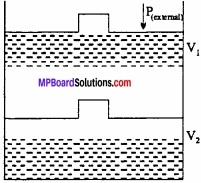Question 12.
An ideal gas filled in a cylinder (according to figure.) is compressed in a single step with external pressure P(external) Then what will be the work done on the gas? Explain with graph?
Solution:
Let the initial volume of the gas is Vi and pressure of cylinder is P. On compressing the gas by pressure P the final volume of gas is Vf.
So, change in volume ∆V = (Vf – Vi)
If W is the work done by the piston on the system
W = P(external) (- ∆V)
W = P(external) (Vf – Vi)
This can be shown in the figure by (P – V) graph, The work done is equal to ABVfVi. The positive sign shows that the work is done on the system.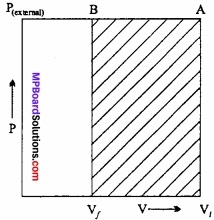Thermodynamics Long Answer Type Questions – I

Question 1.
What is heat capacity? Deduce the expression Cp – Cv = R?
Heat Capacity: It is equal to the amount of heat required to raise the temperature of the system through 1°C. Its unit is JK-1.
Relationship between Cp and Cv:
At constant volume: qv = Cv∆T = ∆U
At constant pressure: qp = Cp∆T = ∆H
∆H and ∆U are related to each other as
∆H = ∆U + ∆ngRT
or ∆H = ∆U + ∆ng(PV)
For 1 mole of ideal gas PV = RT
∴ ∆H = ∆U + ∆(RT)
or ∆H = ∆U + R∆T
on putting the value of ∆H and ∆U
Cp∆T = Cv∆T = Cv∆T + R∆T
Dividing whole equation by ∆T,
Cp = Cv + R
Cp – Cv = R
Thus, value of Cp is always more than Cv and the difference between them is about 2 calories or 8.314 joule.
This relationship is known as Meyer’s relationship.
The ratio Cp/Cv:
The ratio of molar heat capacities at constant pressure (Cp) to that at constant volume (Cv) is represented by γ. Value of γ gives information about the atomicity of the gas. Thus,
For monoatomic gases γ = 1.67
For diatomic gases γ = 1.40
For triatomic gases γ = 1.30Question 2.
Explain Enthalpy of combustion? Write its uses also?
Enthalpy of combustion:
The enthalpy change taking place when one mole of substance is completely oxidised or burnt in presence of excess of oxygen is known as enthalpy of combustion. For example, the enthalpy of combustion of methane is 890.4 kJ.
CH4(g) + 2O2(g) → CO2(g) + 2H2O(g) ∆Hc = – 890.4 kJ

Carbon on the other hand is oxidised to carbon monoxide and carbon dioxide.
C(s) + $$\frac{1}{2}$$ O2(g) → CO(g); ∆H = – 110.5kJ
and C(s) + O2(g) → CO2(g); ∆Hc = – 393.5 kJ

In this case enthalpy of combustion of carbon is – 393.5 kJ and not – 110.5 kJ as formation of CO is a result of incomplete combustion of carbon.
Uses of Enthalpy of combustion:

1. To determine the calorific value of fuel.
2. To determine the enthalpy of reaction of compounds.
3. Determination of structure of compounds.
4. To calculate the calorific value of food.

Question 3.
Prove that qr = ∆Hp?
Or, Prove that at constant pressure and constant temperature the heat of reaction is equal to the change in enthalpy of the system?
Suppose enthalpy, internal energy and volume of a system in initial state are H1, U1 and V1 respectively and after gaining heat these values becomes H2, U2 and V2 respectively, then according to definition.
H1 = U1 + PV1, (in initial state) ……………… (1)
H2 = U2 + PV2, (in final state) …………….. (2)
Subtracting eqn. (1) from eqn eqn. (2),
H2 – H1 = U2 – U1 + P(V2 – V1)
or ∆H = ∆U + P∆V
Where ∆H is enthalpy change, ∆U is change in internal energy and ∆V is change in volume. Therefore at constant pressure enthalpy change is equal to sum of internal energy change and expansion type of mechanical work.
According to first law of thermodynamics,
∆U = q – P∆V
q = ∆U + P∆V
= (U2 – U1) + P(V2 – V1)
= (U2 + PV2) – (U1 + PV1)
= H2 – H1
= ∆H
∴ ∆H = qp
Thus, enthalpy change represents the heat change occurring at constant temperature and pressure. It is noteworthy that though q is path dependent, qp is not because ∆H is a state function.Question 4.
Differentiate between Reversible and Irreversible processes?
Differences between Reversible and Irreversible process:
Reversible process:

1. It is carried out infinitesimally slowly i.e., the difference between driving force and the opposing force is very very small.
2. It is an ideal process requiring infinite time for completion.
3. Equilibrium is not disturbed at any stage during the process.
4. Work obtained is maximum.
5. It is an imaginary process and cannot be realised in actual practice.

Irreversible process:

1. This process is carried out rapidly /.e.,the difference between driving force and the opposing force and the opposing force is quite large.
2. It is a spontaneous process requiring finite time for completion.
3. Equilibrium may occur only after the completion of the process.
4. Work obtained is not maximum.
5. It is a natural process which occurs in
6. particular direction under given set of conditions.

Question 5.
What are the factors affecting enthalpy of reaction?
Factors on which enthalpy of a reaction (∆H) depends: Enthalpy of a reaction i.e., ∆H depends upon the following factors:
1. Physical state of reactants and products:
Enthalpy of reaction is affected by the physical state of reactants and products because latent heat of substance is also involved. For example, value of enthalpy of reaction for the formation of liquid water and water vapour is different.
H2(g) + $$\frac{1}{2}$$ O2(g) → H2O(l); ∆H = – 286 kJ
H2(g) + $$\frac{1}{2}$$ O2(g) → H2O(l); ∆H = – 249 kJ

2. Quantities of the reactants involved:
Enthalpy of reaction is affected by the physical state of reactants and products because latent heat of substance is also involved. For example, value of enthalpy of reaction for the formation of liquid water and water vapour is different.
H2(g) + $$\frac{1}{2}$$ O2(g) → H2O(l); ∆H = – 286 kJ
H2(g) + $$\frac{1}{2}$$ O2(g) → H2O(l); ∆H = – 249 kJ

3. Allotropic modifications:
Allotropes of an element may have different enthalpies. For example, enthalpy change during combustion of graphite and diamond is – 393.5 kJ/mol-1 and – 395.4 kJ/mol-1 respectively.
C(graphite) + O2(g) → CO2(g); ∆H = – 393.5 kJ
C(diamond) + O2(g) → CO2(g); ∆H = – 395.4 kJ

4. Temperature:
Value of enthalpy of reaction is dependent on the temperature at which the reaction is carried out. For example, at 25°C enthalpy of formation of HCl(g) is 184.6 kJ while at 75°C it is 184.4 kJ.
H2(g) + Cl2(g) → 2Hl(g); ∆H = 184.6 kJ at 25°C
H2(g) + Cl2(g) → 2HCl(g); ∆H = 184.4 kJ at 75°CQuestion 6.
Prove that at constant volume qv = ∆U?
When a reaction occurs at constant volume, then no work is done by the system. So W = 0.
So, ∆U = q + W
On putting the value, ∆U = q
So, at constant volume the energy is absorbed which increases the internal energy of the system. So,
∆U = q + W = q + p∆V, (W = P∆V)
Since, the reaction occurs at constant volume. So, ∆V = 0.
On putting vallue, ∆U = qv

Question 7.
From following data determine the heat of reaction of CH4 or enthalpy, ∆H:
C(s) + O2(g) → CO2; ∆H = – 97k cal ………… (1)
2H2(g) + O2(g) → 2H2O(g) ∆H = – 136k cal …………. (2)
CH4 + 2O2(g) → CO2(g) + 2H2O(g); ∆H = – 212k cal ………………… (3)
Solution:
To determine
C(s) + 2H2(g) → cH4(g); ∆H = ?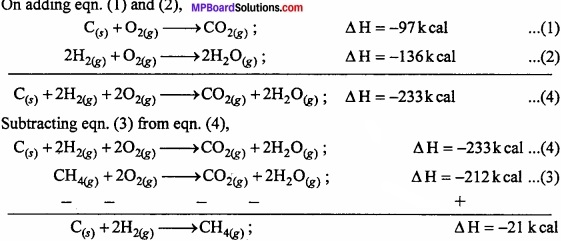Thermodynamics Long Answer Type Questions – II

Question 1.
What is Hess’s Law of constant heat summation? Explain with an example?
In 1840, G.H. Hess gave an important law of constant heat summation according to which, “The enthalpy change in a particular reaction is always constant and does not depend on the path in which reaction takes place”.
Or
“The enthalpy change in a physical or chemical process is the same whether the process is carried out in one or in several steps.”
This law is based on the law of conservation of energy. Suppose that the conversion of substance A to (MPBoardSolutions.com) substance Z takes place in a single step by first method and through several steps in second method. In single step by first method and through several steps in second method. In single step:
A → Z + Q1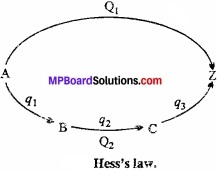Where, Q1 is the energy in several steps:
A → B + q1
B → C + q2
C → Z + q3
Total energy evolved in several steps = q1 + q2 + q3
= Q2 calories (suppose)
Acoording to Hess’s law,
Q1 = Q2
Suppose Hess’s law is incorrect and Q2 > Q1. In this stage if we convert A to Z by several steps and then Z directly to A, then heat equal to (Q2 – Q1) is produced. By repeating this cyclic process several times, an (MPBoardSolutions.com) unlimited amount of heat (energy) may be produced in an isolated system. But this is against the law of conservation of energy.
Practically also, Hess’s law is proved to be true.
Example: Carbon can be directly burnt to produce C02 or in the second method it is first converted to carbon monoxide and then oxidized to carbon dioxide.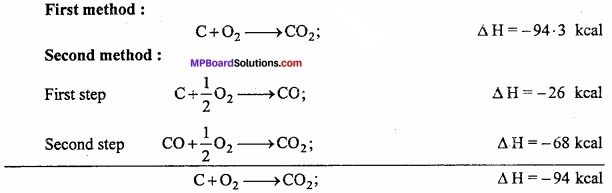Energy evolved by both the methods is nearly same. Different of 0.3 kcal is due to experimental error.
∴ ∆H = ∆H1 + ∆H2

Question 2.
Prove that ∆H = ∆U + P∆V?
Or, Explain the relationship between ∆H and ∆U?
Relation between AH and AU: In case of solids and liquids, the difference between ∆H and ∆U is not significant but in gases it is significant. Let us consider a reaction involving gases. Let the process be isothermal and carried out at constant pressure (P). If VA is the total volume of the gaseous reactants and VB be the total volume of gaseous products, also nA be the number of moles of gaseous reactants and nB be the number of moles of gaseous products. Then
PVA = nART ……………………. (1)
and PVB = nBRT ………………….. (2)
Substarcting eqn. (1) from eqn. (2) we get
PVB – PVA = (nB – nA)RT
or P(VB – VA) = (nB – nA)RT
P∆V = ∆nRT
For gaseous reactants PVR = nRRT …………………… (3)
and for gaseous products PVp = npRT …………………… (4)
Substracting eqn. (3) from eqn. (4), we get
P(Vp – VR) = (np – nR) RT
or P∆V = ∆ngRT ……………………. (5)
But enthalpy change ∆H = ∆U + P∆V …………………….. (6)
Substracting the value of P∆V from eqn. (5) into eqn. (6), we get
∆H = ∆U + ∆ngRT ………………. (7)
Thus, using above eqn. (7) ∆H can be converted into ∆U or vice – versa.eqn. (7) can be wriien as
qp = qv + ∆ngRT ………………….. (8)
Because ∆H = qp and ∆U = qv.
Conditions:

1. If the number of moles of products is greater than that of reactants than ∆n will be +ve and ∆H is greater than ∆U. So,
∆H = ∆U + ∆nRT

2. If the number of moles of reactants is greater than the number of moles of products then ∆n will be – ve and the value of ∆H is less than ∆U.
∆H = ∆U – ∆nRT

3. If the No. of moles of reactants is equal to No. of moles of products then ∆n = 0, then in this condition ∆H = ∆U.Question 3.
Deduce an expression for PV work done?
Let us, consider a cylinder, fitted with a weightless, frictionless piston having a cross – sectional area A, filled with 1 mole of an ideal gas. The total volume of gas is Vi and pressure inside the cylinder is Pin.
Suppose, external pressure on the gas is Pex which is slightly greater than the internal pressure of the gas. (MPBoardSolutions.com) Due to this difference in pressure the gas is compressed till the pressure inside becomes equal to Pex Suppose, the change is achieved in one single step and the final volume of the gas is Vf The gas is compressed and suppose the piston moves a distance l. Work done during compression is
W = Force × Displacement = F × l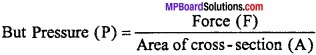or F = P × ABV
∴W = P × A × large
or W = – P∆V, [∴ A × l = ∆V]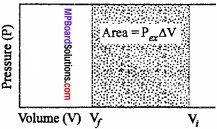The negative sign in the expression is required to obtain conventional sign W. In compression, Vf < Vi and therefore, (Vf – Vi) or ∆V is – ve.
Hence, W will come out to be +ve from the above expression. In expansion type work Vf > Vi and value of ∆V is positive, therefore, work done will be negative.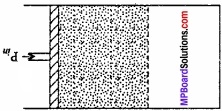This expression is useful for all types of PVwork and for irreversible flow.
Now, we will calculate, the work done during expansion of ideal gas in a reversible manner and in isothermal condition.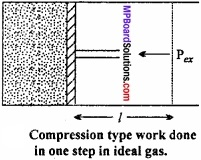Question 4.
What is free energy? Derive its mathematical form and write Gibbs – Helmholtz equation?
The free energy of a system is defined as the maximum amount of energy of the system which can be converted into useful work.
Free energy is related to enthalpy (H), entropy (S) and absolute temperature (T) as,
G = H – TS
Since, we know H = E + PV
∴ G = E + PV – TS
Change in free energy may be given as,
∆G = ∆E + ∆(PV) – ∆(TS)
If the process is carried out at constant temperature and pressure, then
∆(TS) = T∆S and ∆(PV) = P∆V
∆GTp = ∆E + P∆V – T∆S or
∆G = ∆H – T∆S
The above equation is called Gibbs – Helmholtz equation and it helps to predict the spontaneity of a process.
Free energy change and spontaneity:
For a system which is not isolated with surroundings
∆Stotal = ∆Ssystem + ∆Ssurrounding …………………… (1)
when reaction takes place at constant temperature and constant pressure, heat is supplied to surrounding.
∆Ssurrounding = $$\frac { -q_{ p } }{ T }$$ = $$\frac { -\Delta H }{ T }$$, (qp = ∆H at constant pressure) ………………………. (2)
From eqns. (1) and (2),
∆Stotal = ∆Ssystem – $$\frac { -\Delta H }{ T }$$ …………….. (3)
Since, all the quantities on the right – hand side are system properties, the subscript ‘system’ is not used in equations.
Multiplying both sides by T, we get
T∆Stotal = T∆S – ∆H, (Where, ∆S = ∆Ssystem)
or -T∆Sc = ∆H – T∆S …………………… (4)
For Gibbs free energy (G),
G = H – TS
∆G = ∆H – T∆S – S∆T ………………… (5)
or ∆G = ∆H – T∆Stotal
For the process taking place at constant temperature and constant pressure, eqn. (5) will be
So that, ∆G = ∆H – T∆S ……………………… (6)
Comparing eqns. (4) and (6),
∆G = – T∆Stotal ………………….. (7)
So that, ∆G = – ve(for sontaneous chnages)
We know that, for spontaneous chemical change ∆Stotal is positive. Eqn. (7) shows that the spontaneity of a change can be predicted on the basis of the value of ∆G.
Three special cases may be considered according to eqn. (7):

1. If AG is negative, the change is spontaneous.
2. If AG is zero, the system is in equilibrium.
3. If AG is positive, the change is non – spontaneous.

Conditions for spontaneity of a process (conditions for ∆G to be – ve):

1. If AH is negative and AS is positive, AG would certainly be negative and the process will be spontaneous.
2. If AH is negative and AS is also negative, then AG would be negative if AH is greater than TAS in magnitude.Question 5.
Explain the determination of internal energy ∆U by Bomb calorimeter under the following heads:

1. Labelled diagram of the apparatus,
2. Explanation of the process
3. Calculations.

Experimental determination of change in internal energy:
The change in internal energy in a chemical reaction is determined with the help of an apparatus called bomb calorimeter. (MPBoardSolutions.com) It is made up of steel so that it can bear high pressure developed during the chemical reaction taking place in the calorimeter. The inner side of the steel vessel is coated with some non – oxidizable metal like Pt or Au. It is also fitted with a pressure tight screw – cap. The two electrodes are connected to each other through a platinum wire dipped in a platinum cup.

A small known mass of the substance under investigation is taken in the platinum cup. The bomb is filled with excess of oxygen under a pressure of 20 – 25 atm and sealed. Now it is kept in an insulated water – bath which contains a known amount of water. The water – bath is also provided with a thermometer and mechanical stirrer.

The initial temperature of water is noted and the reaction (i.e., combustion of the sample) is started by passing an electric current through the Pt wire. (MPBoardSolutions.com) The heat evolved during the chemical reaction raises the temperature of water which is recorded by the thermometer. When rise in temperature and the heat capacity of the calorimeter are known, the amount of heat evolved in the chemical reaction can be calculated. This will be equal to the change in internal energy (∆E) of the reaction.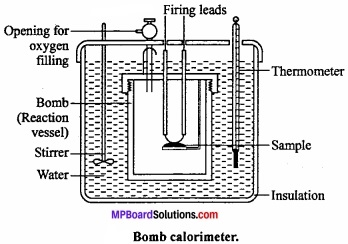Calculation: Let W = Mass of calorimeter in gm, w = Water equivalent of calorimeter, bomb stirrer, etc. t°C = Rise in temperature, x = Mass of compound ignited in gm and m = Molecular mass of the compound.
Heat produced by x gm compound = (W + w) t calories
∴ Heat of combustion of the compound at constant volume,
∆U or ∆E = $$-\frac { m }{ x }$$ (W + w) t calorie/mol.
Heat is evolved so negative sign is used.
Using the equation ∆H = ∆U + ∆ng RT heat of combustion ∆H at constant pressure can be caluculated.